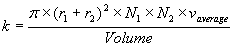## Enzyme - substrate velocity simulator

You must have a Java enabled browser to use this module!

This second simulator uses the same technology shown in the first page in 2 dimensions. However, now the particles are not shown and we are using a three dimensional model. The purpose of this second applet is to demonstrate that for three dimensions a probabilistic model (predicting the line shown in red) quite closely approximates simulating the movement of particles in three dimensions (simulated in blue). The expected number of collisions in the hard sphere model can be calculated using:With r1 and r2 being the radii of the molecules colliding, N1 and N2 are the number of each in the Volume, and v(average) is the average velocity of the particles. Whenever the program above is started by e.g. going to this page or hitting "Refresh" in your browser a simulated box is created and filled with enzyme and substrate at definite concentrations. A background thread which models the movement of all the molecules of both enzyme and substrate is started. You can manually turn the simulation on and off using the corresponding buttons. You can also change the radii and the speeds of the enzyme spheres. As the substrate concentration increases the amount of computing time increases as well considerably slowing this simulator. Luckily, as you can see in red, the average expected number of collisions models the data quite well allowing us to use this as a surrogate for tracking the movement of each particle. The assumptions only apply to a range of speed and radius values and you can see at very large or small enzyme radii or very high or very low speeds the assumptions of the simulation break down and the predicted line deviates from the best fit. A completed example of this kind of simulation is shown below using different particle diameters and speeds. The blue points are the results of the simulation while the line indicates the most likely number of collisions modeled by the equation above.Enzyme Tutorial 2 by Darrick Carter; Copyright 2003, Proteinchemist.com